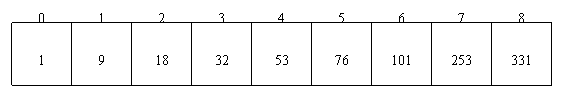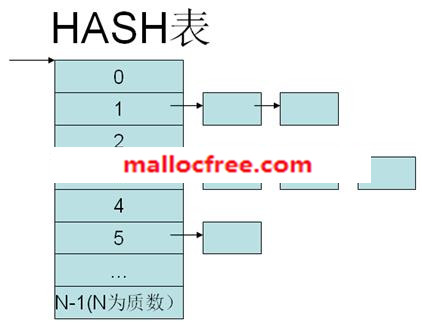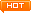﻿ 第七章：查找

## 7.1折半查找//a为存放数据的有序表，n为数据元素个数，k为要查找的元素

int BinSearch(int a[], int n, int k)

{

int low, high, mid, find, i;

find = 0;

low = 0;

high = n-1;

while (low <= high && !find)

{

mid = (low + high)/2;

if (a[mid] < k)

low = mid + 1;

else if (a[mid] > k)

high = mid - 1;

else

{

i = mid;

find = 1;

}

}

if (!find)

i = -1;

return i;

}

int IterBiSearch(int data[], const int x, int beg, int last)

{

int mid = -1;

mid = (beg + last) / 2;

if (x == data[mid])

{

return mid;

}

else if (x < data[mid])

{

return IterBiSearch(data, x, beg, mid - 1);

}

else if (x > data[mid])

{

return IterBiSearch(data, x, mid + 1, last);

}

return -1;

int BinSearch(int a[], int n, int k)

{

return  IterBiSearch(a,k,0,n-1);

}

## 7.2Hash查找

Hash表用于存放key-value数据。比如一个学生的成绩，那么学生的学号可以当做key，成绩当做value，存放与hash表中。Hash查找必须提供一个Hash函数，用于通过Key来计算数据存放在hash表中的位置。一般hash函数可以设计为key%N，其中Nhash表中元素的个数（一般为质数）。假如HASH表的大小为N，那么Hash函数为：

Hash(key)=key%N

int hash(char ch)

{

return ch;

}

char find_first_norepeat_ch(const char *str)

{

int hasharr={0};

char *s=(char *)str;

while(*s)

{

hasharr[hash(*s)]++;

s++;

}

s=(char *)str;

while(*s)

{

if(hasharr[*s]==1)

{

return *s;

}

s++;

}

return '\0';

}

## 7.3二叉搜索树查找

struct _node

{

int data;

struct _node *left;

struct _node *right;

} node, btree;

btree *search(btree *b, int x)

{

if (b == NULL)

{

return NULL;

}

else

{

if (b->data == x)

{

return b;

}

else if (x < b->data)

{

return (search(b->left));

}

else

{

return (search(b->right));

}

}

}看文字不过瘾？点击我，进入周哥教IT视频教学